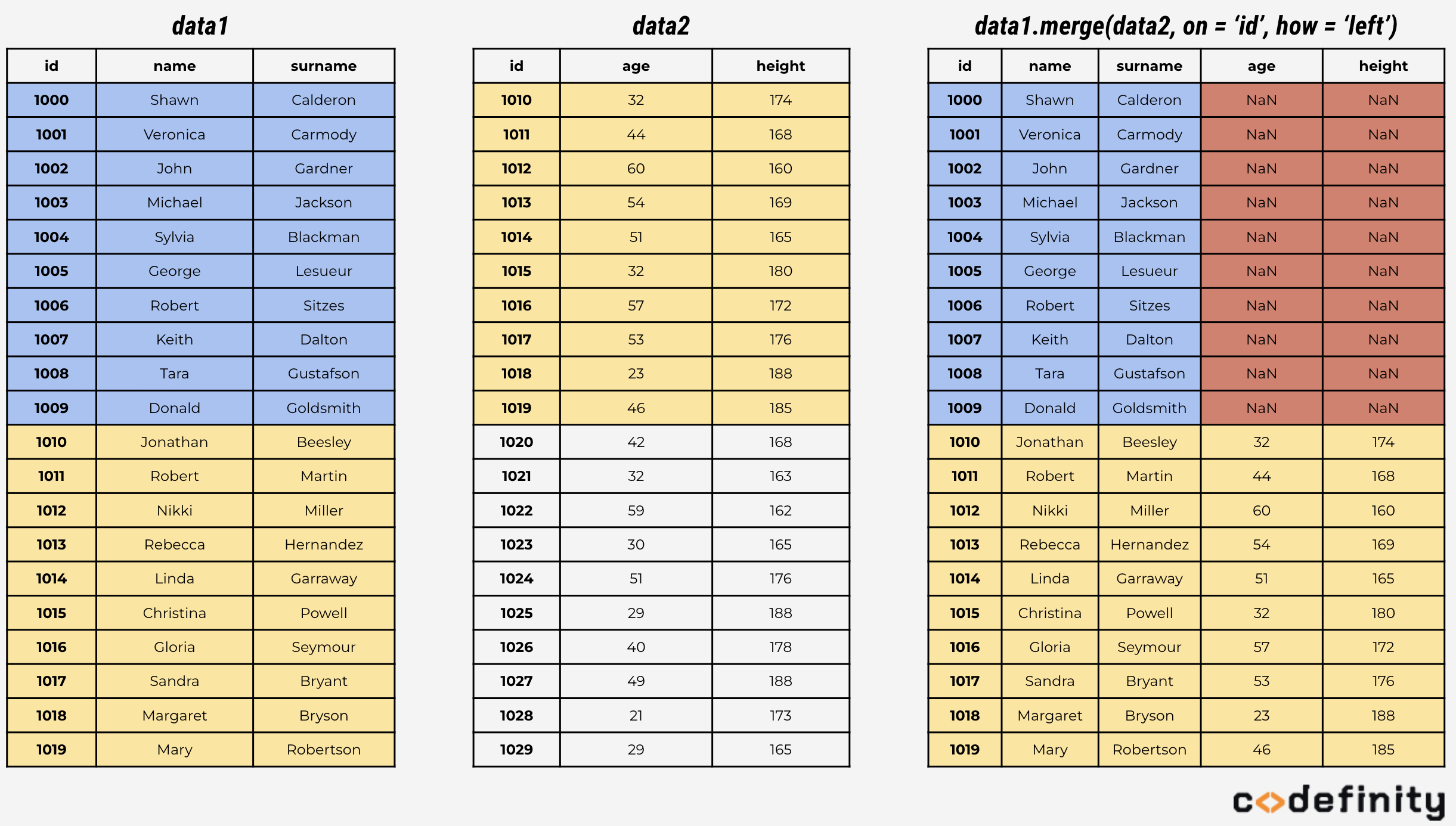Course Content

# Data Manipulation using pandas

Data Manipulation using pandas

##Left Join

Let's start with left and right joins. What does left join mean? Left join returns all the records from the left table, and matching records from the right table. If not all the records were matched, than remaining fields would be filled with NAs.

## Implementation in Python

All types of joins are implemented in `pandas` with the `df_left.merge(df_right, on = 'column', how = 'method')` function. There `df_left` is the left table, `df_right` is the right one, `on` - key field (column) that will be used for comparison, `how` - type of join.

For instance, let's perform a left join of two dataframes. Column with unique values in each dataframe is `id`. To perform a left join, set the `how = 'left'` parameter.As you can see, all the records from the `data1` table were left, and only matching record from the `data2` table were added.

Everything was clear?

Section 5. Chapter 2